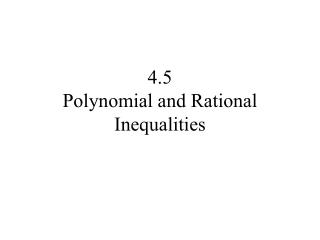DownloadDownload Presentation4.5 Polynomial and Rational Inequalities

# 4.5 Polynomial and Rational Inequalities

Télécharger la présentation## 4.5 Polynomial and Rational Inequalities

- - - - - - - - - - - - - - - - - - - - - - - - - - - E N D - - - - - - - - - - - - - - - - - - - - - - - - - - -
##### Presentation Transcript

1. 4.5 Polynomial and Rational Inequalities

2. Write the inequality in one of the following forms: Steps for Solving Polynomial and Rational Inequalities Algebraically where f(x) is written as a single quotient. • Determine the numbers at which f(x) equals zero and also those numbers at which it is undefined.

3. Use these numbers to separate the real line into intervals. • Select a test number from each interval and evaluate f at the test number. • (a) If the value of f is positive, then f(x)> 0 for all numbers x in the interval. • (b) If the value of f is negative, then f(x)<0 for all numbers x in the interval. If the inequality is not strict, include the solutions of f(x)=0 in the solution set, but do not include those where f is undefined.

4. Solve the inequality: The inequality is in lowest terms, so we will first find where f(x)=0. And where is it undefined. Undefined for x=-2

5. The real line is split into:

6. Pick x =-3 Pick x =0 Pick x =2 Pick x =-3/2 f(-3)=-8 f(-3/2)=5/2 f(0)=-1/2 f(2)=3/4 POSITIVE NEGATIVE POSITIVE NEGATIVE The solution is all numbers x for which or